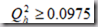﻿ PLS偏最小二乘回归原理与应用-一起大数据

# PLS偏最小二乘回归原理与应用

### 一、前言

##### 2.回归是什么?

”回归“一词来源于对父母身高对于子女身高影响的研究。有人对父母的身高与子女身高做统计，发现除了父母高则子女普遍高的常识性结论外，子女的身高总是“趋向”于人类平均身高，最早“回归”一词就来源于此，即子女的身高总是回归于人类平均身高。

##### 3.回归的方法有哪些?

• 多元线性回归：入门练习常见，但用在模型上基本无用，因为多个变量之间难免有复杂的相关性，多元线性回归不能处理多个自变量之间的“糅合”性。
• 逻辑回归：当预测的是1/0时使用。 这也是机器学习中的一种常用二分类方法。
• 主成分回归：类似于主成分分析，将具有相关性的多维变量降维到互不相关的少数几维变量上，可以处理变量之间具有相关性的情况。
• 岭回归：同上，但方法不同。

• 偏最小二乘回归：当数据量小，甚至比变量维数还小，而相关性又比较大时使用，这个方法甚至优于主成分回归。

4.回归的检验？

• 自变量与因变量是否具有预期的关系。如果有非常不符合逻辑的系数，我们就应该考虑剔除它了。
• 自变量对模型是否有帮助。如果自变量的系数为零（或非常接近零），我们认为这个自变量对模型没有帮助，统计检验就用来计算系数为零的概率。如果统计检验返回一个小概率值（p值），则表示系数为零的概率很小。如果概率小于0.05，汇总报告上概率（Probability）旁边的一个星号（*）表示相关自变量对模型非常重要。换句话说，其系数在95%置信度上具有统计显著性。
• 残差是否有空间聚类。残差在空间上应该是随机分布的，而不应该出现聚类。
• 模型是否出现了倾向性。如果我们正确的构建了回归分析模型，那么模型的残差会符合完美的正态分布，其图形为钟形曲线。
• 自变量中是否存在冗余。建模的过程中，应尽量去选择表示各个不同方面的自变量，也就是尽量避免传达相同或相似信息的自变量。 评估模型的性能。评估矫R2值，有时还要加上修正的Akaike信息准则/Akaike’s information criterion (AIC)，效果是否好。

### 二、进入正题：偏最小二乘回归

##### 1.偏最小二乘回归的思想：

2.偏最小二乘回归建模原理：

(1) t1和u1应尽可能大地携带他们各自数据表中的变异信息;

(2) t1与u1的相关程度能够达到最大。

3.推导偏最小二乘回归：

###### 因此，综合上述两点，我们只要要求t1与u1的协方差达到最大，即：

Cov(t1,u1) –> max

max <E0w1,F0c1>

S.T.

||W1|| = 1;

||c1|| = 1

###### 建立E0，F0对t1，u1的三个回归方程，如下：###### 用残差矩阵E1和F1取代E0和F0,然后,求第二个轴w2和c2以及第二个成分t2,u2,有###### 如此经过step3-step4反复，若E0的秩为A，则可以求出：Fak为残差矩阵Fa的第k列。这样，就求出了回归方程。

###### 这是最后一步，也是非常重要的一步。下面要讨论的问题是在现有的数据表下,如何确定更好的回归方程。在许多情形下,偏最小二乘回归方程并不需要选用全部的成分进行回归建模,而是可以象在主成分分析一样,采用截尾的方式选择前m 个成分,仅用这m 个后续的成分就可以得到一个预测性较好的模型。事实上,如果后续的成分已经不能为解释因变量提供更有意义的信息时,采用过多的成分只会破坏对统计趋势的认识,引导错误的预测结论。(2) 对于k=1,2,…,q,至少有一个k,使得。这时增加成分th,至少使一个因变量yk的预测模型得到显著的改善,因此,也可以考虑增加成分th是明显有益的。

上面推导了偏最小二乘回归，分析了其中的原理。为了使得在实际应用中更加快速的使用偏最小二乘回归，在此，贴上实现偏最小二乘法实现的简洁步骤，需说明的是，下面算法来自司守奎老师《数学建模算法与应用》一书，该书推导过程跨度大，个人认为不适合新手直接阅读，建议你在理解了上述第二部分后再去阅读此书“偏最小二乘回归”章节，定会有更加高层次的认识。步骤如下：有必要贴出偏最小二乘的简单建模应用，并用matlab去是实现之，你可以按照上述步骤，通过基本的运算如求矩阵特征值等，来实现，也可以使用matlab工具箱方法实现之，下面给出的依旧是一个来自司守奎老师书本上的案例：```clc,clear
mu=mean(pz);sig=std(pz); %求均值和标准差
rr=corrcoef(pz); %求相关系数矩阵
data=zscore(pz); %数据标准化,变量记做 X*和 Y*
n=3;m=3; %n 是自变量的个数,m 是因变量的个数
x0=pz(:,1:n);y0=pz(:,n+1:end); %原始的自变量和因变量数据
e0=data(:,1:n);f0=data(:,n+1:end); %标准化后的自变量和因变量数据
-679-
num=size(e0,1);%求样本点的个数
chg=eye(n); %w 到 w*变换矩阵的初始化
for i=1:n
%以下计算 w，w*和 t 的得分向量，
matrix=e0'*f0*f0'*e0;
[vec,val]=eig(matrix); %求特征值和特征向量
val=diag(val); %提出对角线元素，即提出特征值
[val,ind]=sort(val,'descend');
w(:,i)=vec(:,ind(1)); %提出最大特征值对应的特征向量
w_star(:,i)=chg*w(:,i); %计算 w*的取值
t(:,i)=e0*w(:,i); %计算成分 ti 的得分
alpha=e0'*t(:,i)/(t(:,i)'*t(:,i)); %计算 alpha_i
chg=chg*(eye(n)-w(:,i)*alpha'); %计算 w 到 w*的变换矩阵
e=e0-t(:,i)*alpha'; %计算残差矩阵
e0=e;
%以下计算 ss(i)的值
beta=t\f0; %求回归方程的系数，数据标准化，没有常数项
cancha=f0-t*beta; %求残差矩阵
ss(i)=sum(sum(cancha.^2)); %求误差平方和
%以下计算 press(i)
for j=1:num
t1=t(:,1:i);f1=f0;
she_t=t1(j,:);she_f=f1(j,:); %把舍去的第 j 个样本点保存起来
t1(j,:)=[];f1(j,:)=[]; %删除第 j 个观测值
beta1=[t1,ones(num-1,1)]\f1; %求回归分析的系数,这里带有常数项
cancha=she_f-she_t*beta1(1:end-1,:)-beta1(end,:); %求残差向量
press_i(j)=sum(cancha.^2); %求误差平方和
end
press(i)=sum(press_i);
Q_h2(1)=1;
if i>1, Q_h2(i)=1-press(i)/ss(i-1); end
if Q_h2(i)<0.0975
fprintf('提出的成分个数 r=%d',i); break
end
end
beta_z=t\f0; %求 Y*关于 t 的回归系数
xishu=w_star*beta_z; %求 Y*关于 X*的回归系数，每一列是一个回归方程
mu_x=mu(1:n);mu_y=mu(n+1:end); %提出自变量和因变量的均值
sig_x=sig(1:n);sig_y=sig(n+1:end); %提出自变量和因变量的标准差
ch0=mu_y-(mu_x./sig_x*xishu).*sig_y; %计算原始数据回归方程的常数项
for i=1:m
xish(:,i)=xishu(:,i)./sig_x'*sig_y(i); %计算原始数据回归方程的系数
end
sol=[ch0;xish] %显示回归方程的系数，每一列是一个方程，每一列的第一个数是常数项
save mydata x0 y0 num xishu ch0 xish```《数学建模算法与引用》司守奎老师

pdf下载链接：

http://vdisk.weibo.com/s/t0L2pU6fgiP9L?category_id=0&parents_ref=t0L2pU6fgiPav